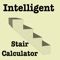See on store
Category
Store
Last Updated
2020, Mar 11
Visibility Score
0

Jun, 2020
Worldwide

Jun, 2020
Worldwide

## Description

For to view How to use https://www.youtube.com/watch?v=x9ilSI_d2GE First GUI ...................... Choice I When you change rise slider It will calculate number of rise through that , it will calculate Total run and It will set Total run Value. When you change run slider It will calculate number of runs through that ,it will calculate Total rise and It will set Total rise Value. Choice II Upside down of Choice One ,When you change rise slider It will calculate number of rise through that ,it will calculate Total rise and It will set Total rise Value. When you change run slider It will calculate number of runs through that ,it will calculate Total run and It will set Total run Value. Choice III Fixed Total Rise and Fixed Total Run This is for the Situation where you have fixed Total rise and Fixed Total run move sliders until you get solution if possible. Fill Total Run and Total rise then For Choice 1 and Choice 2 Move sliders It will calculate and even if you click button. For 3d Choice It will be annoying It will for fixed Total rise and total run. It will pop up error Message Until it find correct fixing value. Accept Inputs (I) Total Run and Rise is in feet One run and One rise is in inches (II) Total Run and Rise is in meter One run and One rise is in cm The Button feet inches button change input mode to meter cm. Second GUI ........................ Fill Total Run , Total Rise assume number of steps set the range Example 3 to 19 fill text box with it Move slider to change number of run after setting preferred numbs Move slide bellow according to your choice whether you are with Fixed total run or Fixed Total rise

## Reviews

#### Try MobileAction free for a week!

No credit card required.